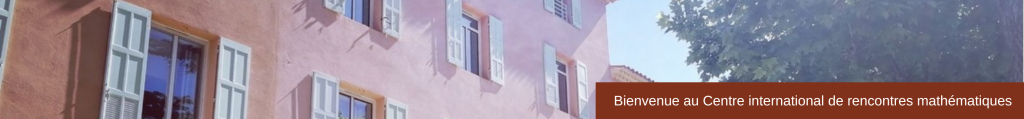Ordered Algebraic Structures and Related Topics
October 12 -16, 2015

Slides or notes

Francesca Acquistapace (Università di Pisa)
A class of globally defined semianalytic sets (pdf)
(joint work with F. Broglia & José F. Fernando Galván)

Vincent Astier (University College Dublin)
(Joint work with Thomas Unger)
Signatures of hermitian forms and orderings on algebras with involution (pdf)

Saugata Basu (Purdue University)
A survey of recent advances in quantitative and algorithmic real algebraic geometry (pdf)

Becker Eberhard (TU Dortmund University) (pdf)

Anna Blaszczok (University of Silesia)
Distances of elements in valued field extensions (pdf)

Greg Blekherman (Georgia Tech)
Sums of Squares on Real Projective Varieties (pdf)

Quentin Brouette (University of Mons)
Stellensatze in closed ordered differential fields (pdf)

On polynomial , regular and Nash images of Euclidean spaces (pdf)
(joint work with J.M. Gamboa & C. Ueno)

(joint work with Murray Marshall)
Witt equivalence of function fields over global fields (pdf)

Charu Goel (University of Konstanz)
The analogue of Hilbert’s 1888 Theorem for even symmetric forms (pdf)

Maria Infusino (University of Konstanz)
Moment problem for symmetric algebras of locally convex spaces (pdf)
(Joint work with M. Ghasemi, S. Kuhlmann and M. Marshall)

﻿Zur Izhakian﻿ (Bar-Ilan University)
Supertropical algebra and representations (pdf)

Franziska Jahnke (WWU Munster)
Dp-minimal ordered fields
joint work with Pierre Simon & Erik Walsberg (pdf)

Tobias Kaiser (Universität Passau)
Lebesgue motivic invariants (pdf)

Katarzyna Kuhlmann (University of Silesia)
Orderings and R-places of function fields (pdf)
(joint work with P. Koprowski)

Franz-Viktor and Katarzyna Kuhlmann
Symmetrically complete ordered fields (pdf)

Henri Lombardi (Université Franche-Comté)
Théories géométriques pour l’algèbre des nombres réels sans test de signe ni axiome de choix dépendant (pdf)

Francisco Miraglia (University of São Paulo, Brazil)
Algebraic Theory of quadratic Forms and Rings (pdf)

Tim Netzer (TU Dresden)
Proving Kazhdan’s Property (T) with Sums of Squares Techniques (pdf)

Daniel Perrucci (Universidad de Buenos Aires)
K ordered field, R real closed field (pdf)

Daniel Plaumann (University of Konstanz)
Positive Polynomials (pdf)

Cordian Riener (Aalto Science Institute)
Topological complexity of symmetric semi-algebraic sets (pdf)
(joint with Saugata Basu)

Bruce Reznick (University of Illinois at Urbana-Champaign)
Ternary forms with lots of zeros (pdf)

Claus Scheiderer (University of Konstanz)
Extremal nonnegative forms (pdf)

Markus Schweighofer (University of Konstanz)
Inclusion of spectrahedra, free spectrahedra and coin tossing (pdf)
(joint work with Bill Helton, Igor Klep and Scott McCullough)

Tamara Servi (Université Paris 7)
Lebesgue integration of oscillating and subanalytic functions (pdf)

Rainer Sinn (Georgia Institute of Technology)
Gram Spectrahedra (pdf)
(with Greg Blekherman (Georgia Tech), Daniel Plaumann (Universität Konstanz) and Cynthia Vinzant (NC State))

Margaret Thomas (University of Konstanz)
Effective Pila-Wilkie bounds for restricted Pfaffian surfaces (pdf)

Grey Violet (University of Konstanz)
Geometry of univariate stability: continuity argument, symmetric products and stability theories (pdf)